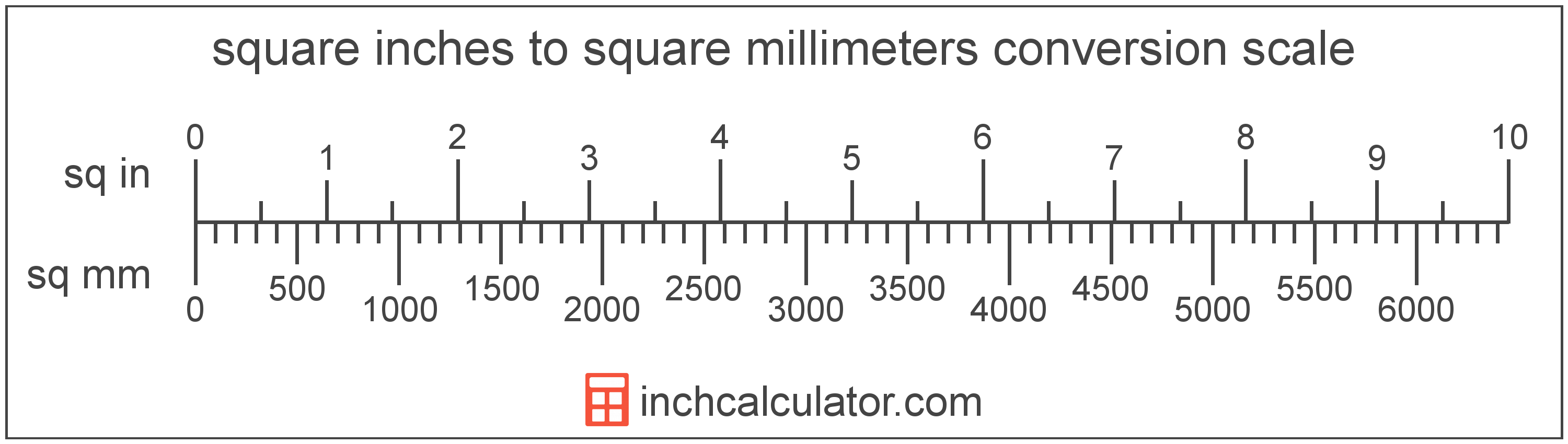# Square Millimeters to Square Inches Converter

Enter the area in square millimeters below to get the value converted to square inches.

Results in Square Inches:1 sq mm = 0.00155 sq in

Do you want to convert square inches to square millimeters?

## How to Convert Square Millimeters to Square Inches

To convert a measurement in square millimeters to a measurement in square inches, multiply the area by the following conversion ratio: 0.00155 square inches/square millimeter.

Since one square millimeter is equal to 0.00155 square inches, you can use this simple formula to convert:

square inches = square millimeters × 0.00155

The area in square inches is equal to the area in square millimeters multiplied by 0.00155.

For example, here's how to convert 500 square millimeters to square inches using the formula above.
square inches = (500 sq mm × 0.00155) = 0.775002 sq in## What Is a Square Millimeter?

One square millimeter is equivalent to the area of a square with edges that are each 1 millimeter in length. One square millimeter is equal to 0.00155 square inches or 1/100 of a square centimeter.

The square millimeter, or square millimetre, is a multiple of the square meter, which is the SI derived unit for area. In the metric system, "milli" is the prefix for thousandths, or 10-3. A square millimeter is sometimes also referred to as a square mm. Square millimeters can be abbreviated as sq mm, and are also sometimes abbreviated as mm². For example, 1 square millimeter can be written as 1 sq mm or 1 mm².

## What Is a Square Inch?

One square inch is equivalent to the area of a square with sides that are each 1 inch in length. One square inch is equal to 1/144 of a square foot or 1/1,296 of a square yard.

The square inch is a US customary and imperial unit of area. A square inch is sometimes also referred to as a square in. Square inches can be abbreviated as sq in, and are also sometimes abbreviated as in². For example, 1 square inch can be written as 1 sq in or 1 in².

## Square Millimeter to Square Inch Conversion Table

Table showing various square millimeter measurements converted to square inches.
Square Millimeters Square Inches
1 sq mm 0.00155 sq in
2 sq mm 0.0031 sq in
3 sq mm 0.00465 sq in
4 sq mm 0.0062 sq in
5 sq mm 0.00775 sq in
6 sq mm 0.0093 sq in
7 sq mm 0.01085 sq in
8 sq mm 0.0124 sq in
9 sq mm 0.01395 sq in
10 sq mm 0.0155 sq in
20 sq mm 0.031 sq in
30 sq mm 0.0465 sq in
40 sq mm 0.062 sq in
50 sq mm 0.0775 sq in
60 sq mm 0.093 sq in
70 sq mm 0.1085 sq in
80 sq mm 0.124 sq in
90 sq mm 0.1395 sq in
100 sq mm 0.155 sq in
200 sq mm 0.310001 sq in
300 sq mm 0.465001 sq in
400 sq mm 0.620001 sq in
500 sq mm 0.775002 sq in
600 sq mm 0.930002 sq in
700 sq mm 1.085 sq in
800 sq mm 1.24 sq in
900 sq mm 1.395 sq in
1,000 sq mm 1.55 sq in